Skip to main content Accessibility help
Home
Hostname: page-component-684bc48f8b-g7stk Total loading time: 1.404 Render date: 2021-04-11T12:41:20.257Z Has data issue: true Feature Flags: { "shouldUseShareProductTool": true, "shouldUseHypothesis": true, "isUnsiloEnabled": true, "metricsAbstractViews": false, "figures": false, "newCiteModal": false, "newCitedByModal": true }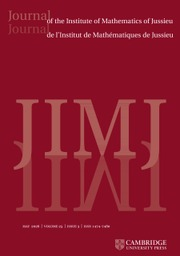Journal of the Institute of Mathematics of Jussieu

# SMOOTH BIMODULES AND COHOMOLOGY OF II1 FACTORS

Published online by Cambridge University Press:  07 May 2015

Corresponding
E-mail address:

## Abstract

We prove that, under rather general conditions, the 1-cohomology of a von Neumann algebra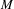$M$ with values in a Banach$M$ -bimodule satisfying a combination of smoothness and operatorial conditions vanishes. For instance, we show that, if$M$ acts normally on a Hilbert space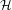${\mathcal{H}}$ and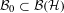${\mathcal{B}}_{0}\subset {\mathcal{B}}({\mathcal{H}})$ is a norm closed$M$ -bimodule such that any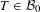$T\in {\mathcal{B}}_{0}$ is smooth (i.e., the left and right multiplications of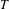$T$ by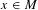$x\in M$ are continuous from the unit ball of$M$ with the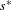$s^{\ast }$ -topology to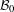${\mathcal{B}}_{0}$ with its norm), then any derivation of$M$ into${\mathcal{B}}_{0}$ is inner. The compact operators are smooth over any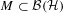$M\subset {\mathcal{B}}({\mathcal{H}})$ , but there is a large variety of non-compact smooth elements as well.

## MSC classification

Type
Research Article
Information
Copyright
© Cambridge University Press 2015

## Access options

Get access to the full version of this content by using one of the access options below.

## References

Akemann, C. E. and Ostrand, P. A., Computing norms in group C -algebras, Amer. J. Math. 98 (1976), 10151047.CrossRefGoogle Scholar
Alekseev, V., On the first continuous$L^{2}$ -cohomology of free group factors. arXiv:1312.5647.Google Scholar
Alekseev, V. and Kyed, D., Measure continuous derivations on von Neumann algebras and applications to$L^{2}$ -cohomology. arXiv:1110.6155.Google Scholar
Bader, U., Gelander, T. and Monod, N., A fixed point theorem for L 1 spaces, Invent. Math. 189 (2012), 143148.CrossRefGoogle Scholar
Bozejko, M., On 𝛬(p) sets with minimal constant in discrete noncommutative groups, Proc. Amer. Math. Soc. 51 (1975), 407412.CrossRefGoogle Scholar
Christensen, E., Derivations and Their Extensions to Perturbations of Operator Algebras, Proceedings of Symposia in Pure Mathematics, Volume 38, pp. 261273 (American Mathematical Society, Providence, RI, 1982).Google Scholar
Connes, A., On the Classification of von Neumann Algebras and Their Automorphisms, Symposia Mathematica, Volume XX, pp. 435478 (Academic Press, London, 1976).Google Scholar
Connes, A. and Shlyakhtenko, D., L 2 -homology for von Neumann algebras, J. Reine Angew. Math. 586 (2005), 125168.CrossRefGoogle Scholar
Dixmier, J., Les algebres d’operateurs dans l’espace hilbertien (Gauthier-Villars, Paris, 1957), 1996.Google Scholar
Dykema, K., Two applications of free entropy, Math. Ann. 308 (1997), 545558.CrossRefGoogle Scholar
Feldman, J. and Moore, C. C., Ergodic equivalence relations, cohomology, and von Neumann algebras I, II, Trans. Amer. Math. Soc. 234 (1977), 289324. 325–359.CrossRefGoogle Scholar
Gaboriau, D., Invariants 2 de rélations d’équivalence et de groupes, Publ. Math. Inst. Hautes Études Sci. 95 (2002), 93150.CrossRefGoogle Scholar
Haagerup, U., All nuclear C -algebras are amenable, Invent. Math. 74 (1983), 305319.CrossRefGoogle Scholar
Ioana, A., Peterson, J. and Popa, S., Amalgamated free products of w-rigid factors and calculation of their symmetry groups, Acta Math. 200 (2008), 85153. arXiv:math.OA/0505589.CrossRefGoogle Scholar
Johnson, B. E., Kadison, R. V. and Ringrose, J. R. , Cohomology of operator algebras III: reduction to normal cohomology, Bull. Soc. Math. France 100 (1972), 7396.CrossRefGoogle Scholar
Johnson, B. and Parrott, S., Operators commuting with a von Neumann algebra modulo the set of compact operators, J. Funct. Anal. 11 (1972), 3961.CrossRefGoogle Scholar
Kadison, R. V. and Ringrose, J. R. , Cohomology of operator algebras I: type I von Neumann algebras, Acta Math. 126 (1971), 227243.CrossRefGoogle Scholar
Kadison, R. V. and Ringrose, J. R., Cohomology of operator algebras II: extended cobounding and the hyperfinite case, Ark. Mat. 9 (1971), 5563.CrossRefGoogle Scholar
Kesten, H., Symmetric random walks on groups, Trans. Amer. Math. Soc. 92 (1959), 336354.CrossRefGoogle Scholar
Kirchberg, E., The derivation problem and the similarity problem are equivalent, J. Operator Theory 36 (1996), 5962.Google Scholar
Murray, F. and von Neumann, J., Rings of operators IV, Ann. of Math. (2) 44 (1943), 716808.CrossRefGoogle Scholar
Peterson, J., L 2 -rigidity in von Neumann algebras, Invent. Math. 175 (2009), 417433.CrossRefGoogle Scholar
Peterson, J. and Thom, A., Group cocycles and the ring of affiliated operators, Invent. Math. 185 (2011), 561592.CrossRefGoogle Scholar
Pisier, G., Factorizations of Linear Operators and the Geometry of Banach Spaces, CBMS Lecture Notes, Volume 60 (1986).CrossRefGoogle Scholar
Pisier, G., Introduction to Operator Space Theory, London Mathematical Society Lecture Notes, Volume 294 (2013).Google Scholar
Pisier, G., Similarity Problems and Completely Bounded Maps, Springer Lecture Notes, Volume 1618 (1995).Google Scholar
Pisier, G., Private communication.Google Scholar
Popa, S., On a problem of RV Kadison on maximal abelian *-subalgebras in factors, Invent. Math. 65 (1981), 269281.CrossRefGoogle Scholar
Popa, S., On derivations into the compacts and some properties of type II factors, in Proceedings of XIV-th Conference in Operator Theory, Herculane–Timisoara 1983 (ed. I. Gohberg), Volume 14, pp. 221–227 (Birkhäuser Verlag, 1984).Google Scholar
Popa, S., The commutant modulo the set of compact operators of a von Neumann algebra, J. Funct. Anal. 71 (1987), 393408.CrossRefGoogle Scholar
Popa, S., Free independent sequences in type II1 factors and related problems, Astérisque 232 (1995), 187202.Google Scholar
Popa, S., Some computations of 1-cohomology groups and construction of non orbit equivalent actions, J. Inst. Math. Jussieu 5 (2006), 309332. arXiv:mathOA/0407199.CrossRefGoogle Scholar
Popa, S., A II1 factor approach to the Kadison–Singer problem, Comm. Math. Phys. (2014). arXiv:1303.1424.Google Scholar
Popa, S., Independence properties in subalgebras of ultraproduct II1 factors, J. Funct. Anal. 266 (2014), 58185846. arXiv:mathOA/1308.3982.CrossRefGoogle Scholar
Popa, S., Classification of amenable subfactors of type II, Acta Math. 172 (1994), 163255.CrossRefGoogle Scholar
Popa, S. and Radulescu, F., Derivations of von Neumann factors into the compact ideal space of a semifinite algebra, Duke Math. J. 57 (1988), 485518.CrossRefGoogle Scholar
Popa, S. and Vaes, S., Vanishing of the continuous first$L^{2}$ -cohomology for II1 factors, Int. Math. Res. Not. IMRN (2014). arXiv:mathOA/1401.1186.Google Scholar
Powers, R. and Størmer, E., Free states of the canonical anticommutation relations, Comm. Math. Phys. 16 (1970), 133.CrossRefGoogle Scholar
Radulescu, F., Vanishing of H w 2(M, K(H)) for certain finite von Neumann algebras, Trans. Amer. Math. Soc. 326 (1991), 569584.Google Scholar
Ringrose, J., Automatic continuity of derivations of operator algebras, J. Lond. Math. Soc. 5 (1972), 432438.CrossRefGoogle Scholar
Sinclair, A. M. and Smith, R. R., Hochschild Cohomology of von Neumann Algebras, London Mathematical Society Lecture Note Series, Volume 203 (Cambridge University Press, Cambridge, 1995).CrossRefGoogle Scholar
Takesaki, M., Theory of Operator Algebras, I, Encyclopaedia of Mathematical Sciences, Volume 124 (Springer-Verlag, Berlin, 2002). Reprint of the first (1979) edition, Operator Algebras and Non-commutative Geometry, 5.Google Scholar
Thom, A., L 2 -cohomology for von Neumann algebras, GAFA 18 (2008), 5170.Google Scholar

### Full text views

Full text views reflects PDF downloads, PDFs sent to Google Drive, Dropbox and Kindle and HTML full text views.

Total number of HTML views: 0
Total number of PDF views: 96 *
View data table for this chart

* Views captured on Cambridge Core between September 2016 - 11th April 2021. This data will be updated every 24 hours.

# Send article to Kindle

To send this article to your Kindle, first ensure no-reply@cambridge.org is added to your Approved Personal Document E-mail List under your Personal Document Settings on the Manage Your Content and Devices page of your Amazon account. Then enter the ‘name’ part of your Kindle email address below. Find out more about sending to your Kindle. Find out more about sending to your Kindle.

Note you can select to send to either the @free.kindle.com or @kindle.com variations. ‘@free.kindle.com’ emails are free but can only be sent to your device when it is connected to wi-fi. ‘@kindle.com’ emails can be delivered even when you are not connected to wi-fi, but note that service fees apply.

Find out more about the Kindle Personal Document Service.

SMOOTH BIMODULES AND COHOMOLOGY OF II1 FACTORS
Available formats
×

# Send article to Dropbox

To send this article to your Dropbox account, please select one or more formats and confirm that you agree to abide by our usage policies. If this is the first time you use this feature, you will be asked to authorise Cambridge Core to connect with your <service> account. Find out more about sending content to Dropbox.

SMOOTH BIMODULES AND COHOMOLOGY OF II1 FACTORS
Available formats
×

# Send article to Google Drive

To send this article to your Google Drive account, please select one or more formats and confirm that you agree to abide by our usage policies. If this is the first time you use this feature, you will be asked to authorise Cambridge Core to connect with your <service> account. Find out more about sending content to Google Drive.

SMOOTH BIMODULES AND COHOMOLOGY OF II1 FACTORS
Available formats
×
×

#### Conflicting interests

Do you have any conflicting interests? *